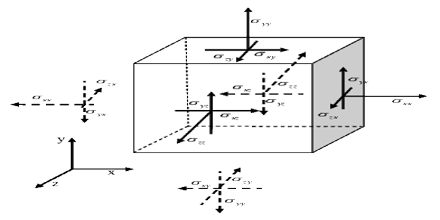Mathematic

# Cartesian Components of VectorsBasic purpose of this lecture is to present on Cartesian Components of Vectors. Any vector may be expressed in Cartesian components by using unit vectors in the directions of the coordinate axes. Here briefly describe Two-dimensional Coordinate frames for understand Cartesian Components of Vectors. In this lecture example; i represents a unit vector in the direction of the positive x-axis and j represents a unit vector in the direction of the positive y-axis.